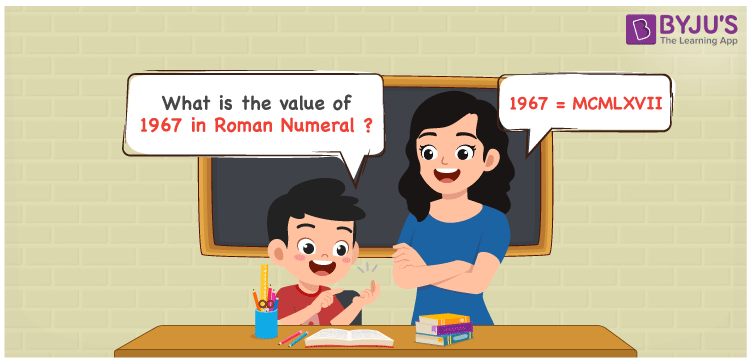# 1967 in Roman Numerals

1967 in Roman Numerals is MCMLXVII. The article on Roman numerals is curated in a way to increase the knowledge of important concepts in Maths among children. The expansion of 1967 according to its place value and its conversion to roman numerals can be understood well here. Hence, 1967 can be written as MCMLXVII in roman numerals.

 Number Roman Numeral 1967 MCMLXVII## How to Write 1967 in Roman Numerals?

For a clear idea of the conversion of 1967 to roman numerals, make use of the steps given below.

1967 = 1000 + (1000 – 100) + 50 + 10 + 5 + 1 + 1

1967 = M + (M – C) + L + X + V + I + I

1967 = MCMLXVII

## Video Lesson on Roman Numerals## Frequently Asked Questions on 1967 in Roman Numerals

Q1

### Write 1967 in Roman Numerals.

1967 in Roman Numerals is MCMLXVII.
Q2

### Calculate 2000 – 33.

We know that

2000 in Roman Numerals = MM

33 in Roman Numerals = XXXIII

As 2000 – 33 = 1967

So 2000 – 33 is 1967 which is written as MCMLXVII.

Q3

### How can 1967 be written in Roman Numerals?

1967 can be written in Roman Numerals considering the ones, tens, hundreds and thousands value of the number.

Thousands = 1000 = M

Hundreds = 900 = CM

Tens = 60 = LX

Ones = 7 = VII

Number = 1000 + 900 + 60 + 7 = M + CM + LX + VII = MCMLXVII